Скачать презентацию CA 208 Logic First-Order Predicate Logic Kate is

066c432de76fef2cf6e4f36a1998ae8e.ppt

• Количество слайдов: 57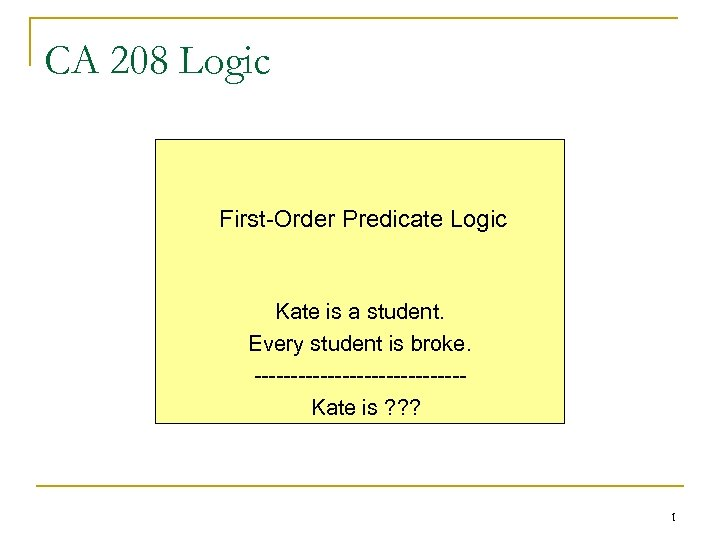CA 208 Logic First-Order Predicate Logic Kate is a student. Every student is broke. --------------Kate is ? ? ? 1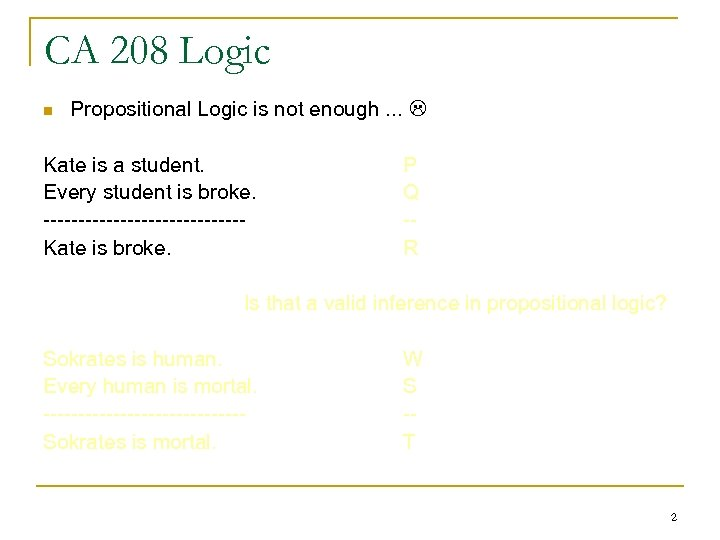CA 208 Logic n Propositional Logic is not enough. . . Kate is a student. Every student is broke. --------------Kate is broke. P Q -R Is that a valid inference in propositional logic? Sokrates is human. Every human is mortal. --------------Sokrates is mortal. W S -T 2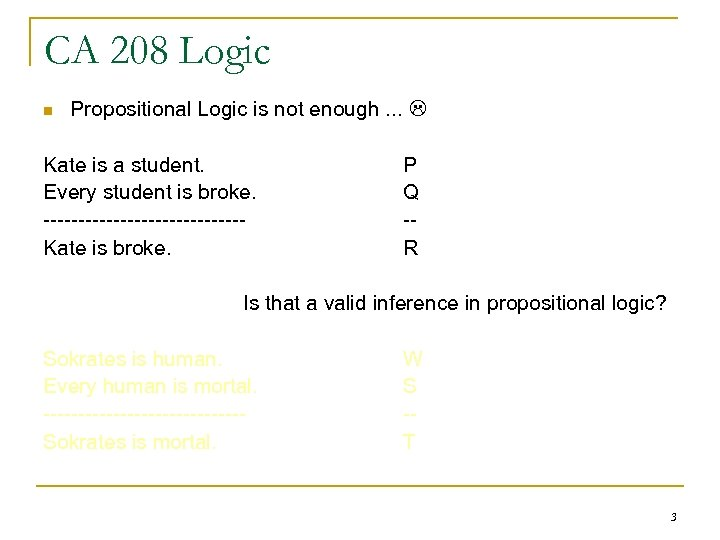CA 208 Logic n Propositional Logic is not enough. . . Kate is a student. Every student is broke. --------------Kate is broke. P Q -R Is that a valid inference in propositional logic? Sokrates is human. Every human is mortal. --------------Sokrates is mortal. W S -T 3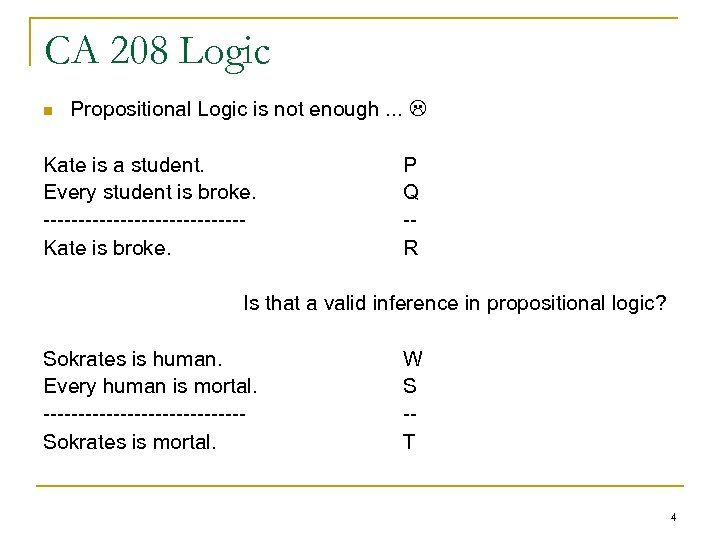CA 208 Logic n Propositional Logic is not enough. . . Kate is a student. Every student is broke. --------------Kate is broke. P Q -R Is that a valid inference in propositional logic? Sokrates is human. Every human is mortal. --------------Sokrates is mortal. W S -T 4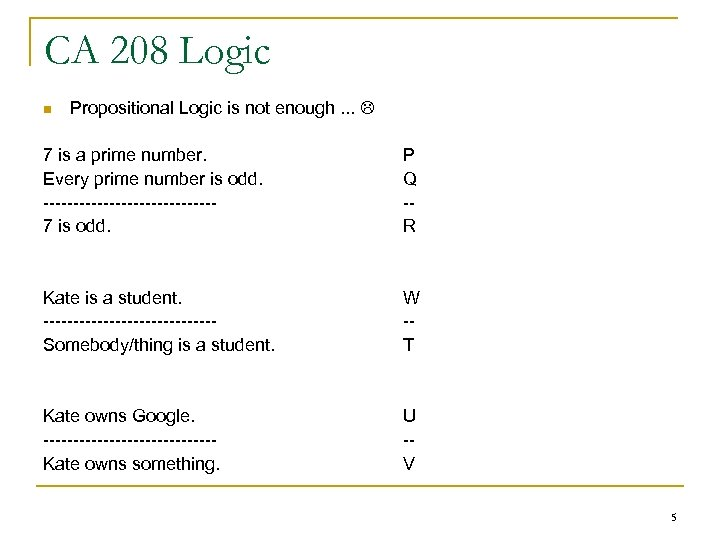CA 208 Logic n Propositional Logic is not enough. . . 7 is a prime number. Every prime number is odd. --------------7 is odd. P Q -R Kate is a student. --------------Somebody/thing is a student. W -T Kate owns Google. --------------Kate owns something. U -V 5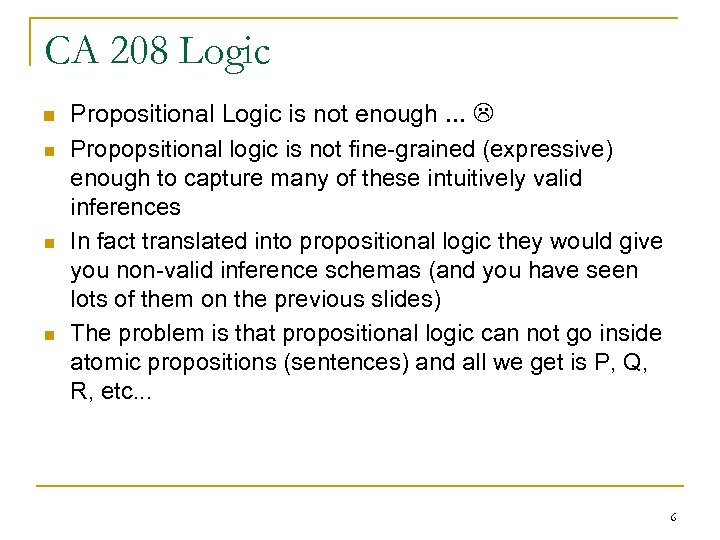CA 208 Logic n n Propositional Logic is not enough. . . Propopsitional logic is not fine-grained (expressive) enough to capture many of these intuitively valid inferences In fact translated into propositional logic they would give you non-valid inference schemas (and you have seen lots of them on the previous slides) The problem is that propositional logic can not go inside atomic propositions (sentences) and all we get is P, Q, R, etc. . . 6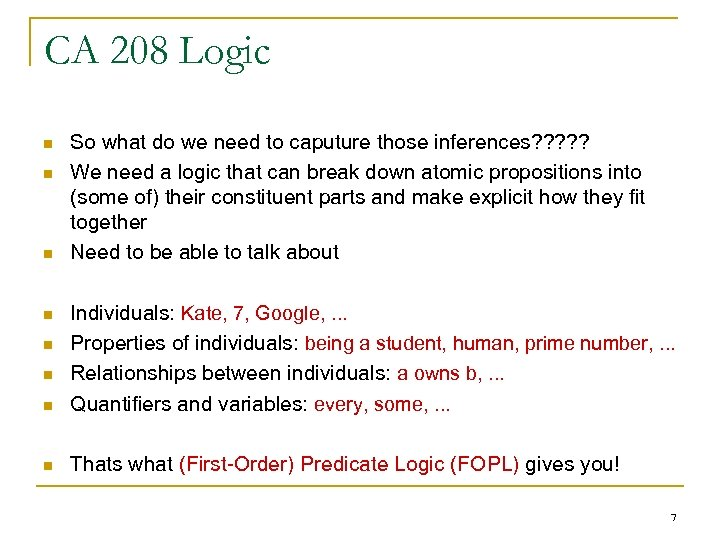CA 208 Logic n n n So what do we need to caputure those inferences? ? ? We need a logic that can break down atomic propositions into (some of) their constituent parts and make explicit how they fit together Need to be able to talk about n Individuals: Kate, 7, Google, . . . Properties of individuals: being a student, human, prime number, . . . Relationships between individuals: a owns b, . . . Quantifiers and variables: every, some, . . . n Thats what (First-Order) Predicate Logic (FOPL) gives you! n n n 7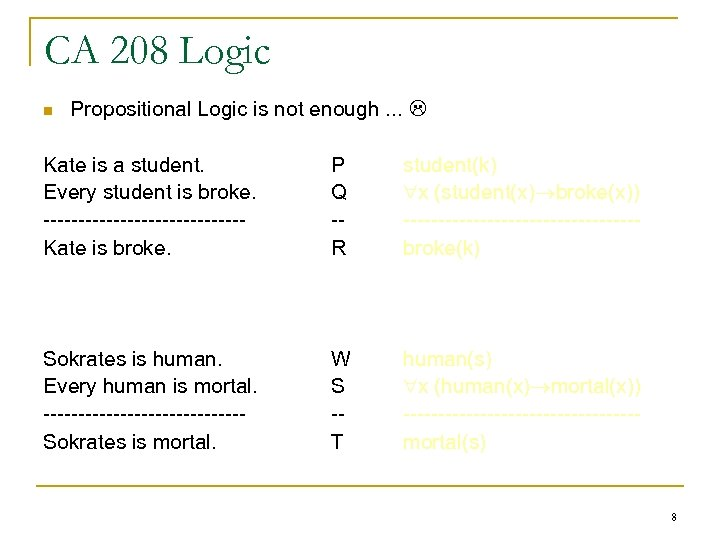CA 208 Logic n Propositional Logic is not enough. . . Kate is a student. Every student is broke. --------------Kate is broke. P Q -R student(k) x (student(x) broke(x)) -----------------broke(k) Sokrates is human. Every human is mortal. --------------Sokrates is mortal. W S -T human(s) x (human(x) mortal(x)) -----------------mortal(s) 8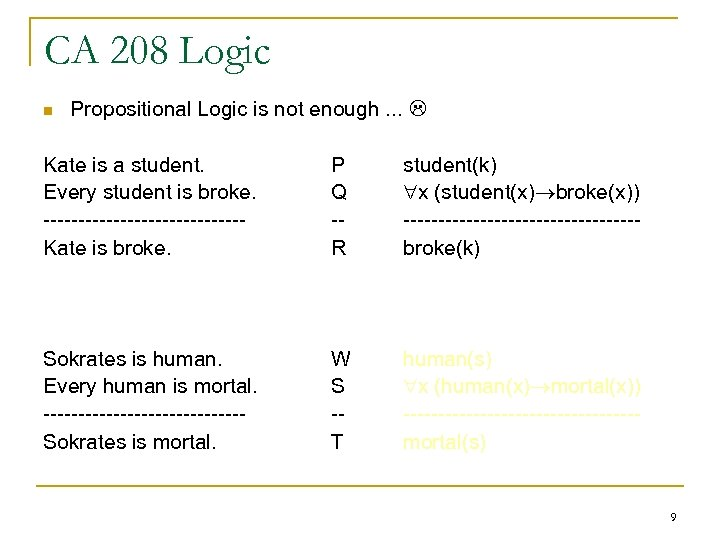CA 208 Logic n Propositional Logic is not enough. . . Kate is a student. Every student is broke. --------------Kate is broke. P Q -R student(k) x (student(x) broke(x)) -----------------broke(k) Sokrates is human. Every human is mortal. --------------Sokrates is mortal. W S -T human(s) x (human(x) mortal(x)) -----------------mortal(s) 9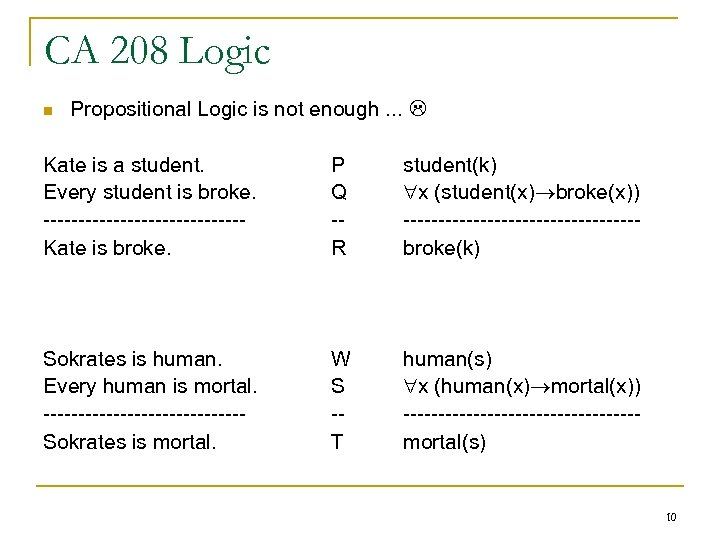CA 208 Logic n Propositional Logic is not enough. . . Kate is a student. Every student is broke. --------------Kate is broke. P Q -R student(k) x (student(x) broke(x)) -----------------broke(k) Sokrates is human. Every human is mortal. --------------Sokrates is mortal. W S -T human(s) x (human(x) mortal(x)) -----------------mortal(s) 10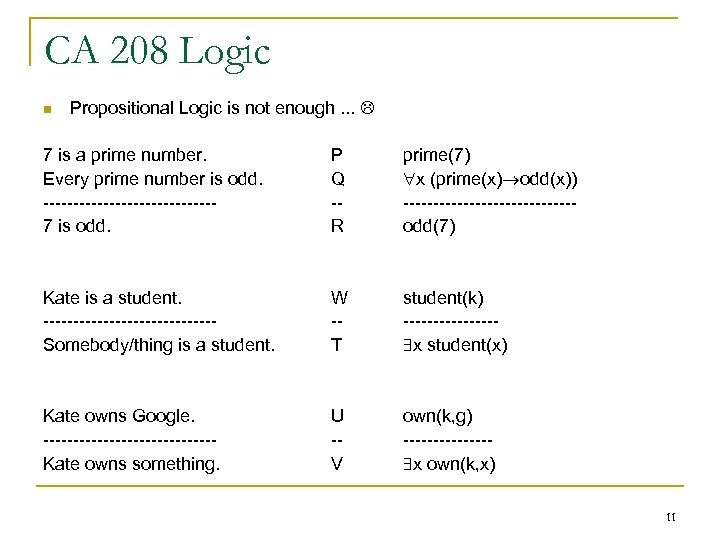CA 208 Logic n Propositional Logic is not enough. . . 7 is a prime number. Every prime number is odd. --------------7 is odd. P Q -R prime(7) x (prime(x) odd(x)) --------------odd(7) Kate is a student. --------------Somebody/thing is a student. W -T student(k) -------- x student(x) Kate owns Google. --------------Kate owns something. U -V own(k, g) ------- x own(k, x) 11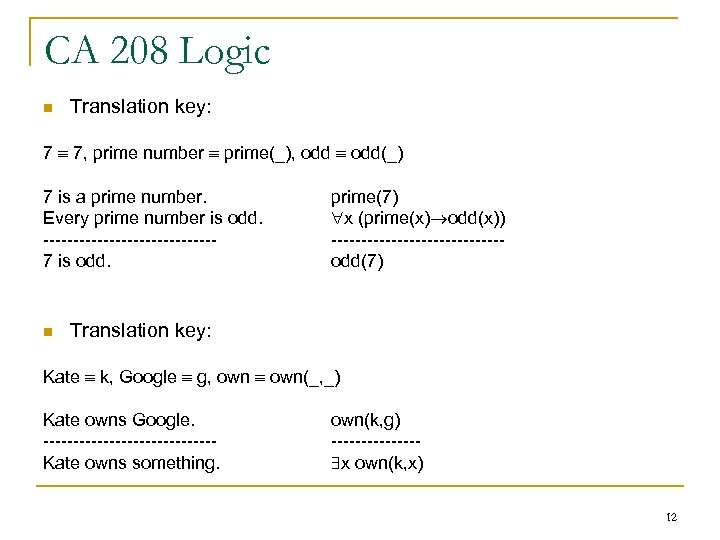CA 208 Logic n Translation key: 7 7, prime number prime(_), odd(_) 7 is a prime number. Every prime number is odd. --------------7 is odd. n prime(7) x (prime(x) odd(x)) --------------odd(7) Translation key: Kate k, Google g, own(_, _) Kate owns Google. --------------Kate owns something. own(k, g) ------- x own(k, x) 12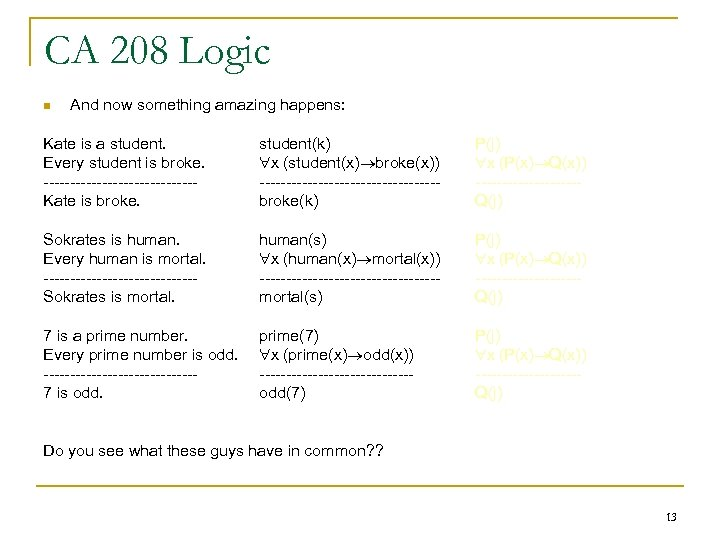CA 208 Logic n And now something amazing happens: Kate is a student. Every student is broke. --------------Kate is broke. student(k) x (student(x) broke(x)) -----------------broke(k) P(j) x (P(x) Q(x)) ----------Q(j) Sokrates is human. Every human is mortal. --------------Sokrates is mortal. human(s) x (human(x) mortal(x)) -----------------mortal(s) P(j) x (P(x) Q(x)) ----------Q(j) 7 is a prime number. Every prime number is odd. --------------7 is odd. prime(7) x (prime(x) odd(x)) --------------odd(7) P(j) x (P(x) Q(x)) ----------Q(j) Do you see what these guys have in common? ? 13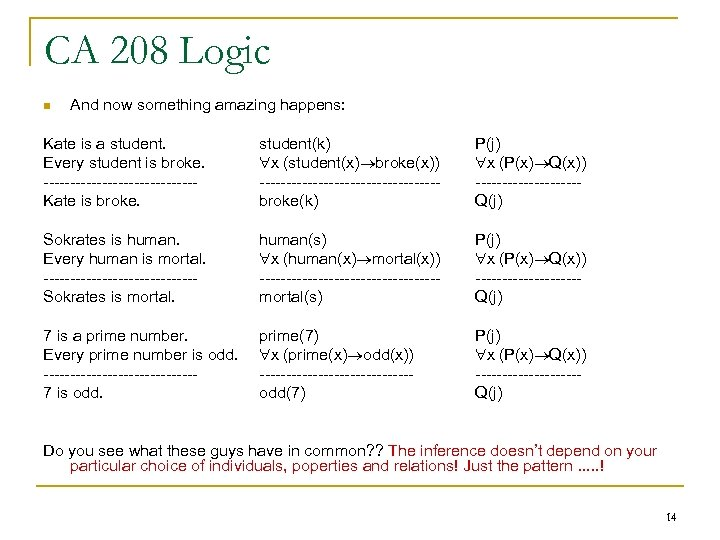CA 208 Logic n And now something amazing happens: Kate is a student. Every student is broke. --------------Kate is broke. student(k) x (student(x) broke(x)) -----------------broke(k) P(j) x (P(x) Q(x)) ----------Q(j) Sokrates is human. Every human is mortal. --------------Sokrates is mortal. human(s) x (human(x) mortal(x)) -----------------mortal(s) P(j) x (P(x) Q(x)) ----------Q(j) 7 is a prime number. Every prime number is odd. --------------7 is odd. prime(7) x (prime(x) odd(x)) --------------odd(7) P(j) x (P(x) Q(x)) ----------Q(j) Do you see what these guys have in common? ? The inference doesn’t depend on your particular choice of individuals, poperties and relations! Just the pattern. . . ! 14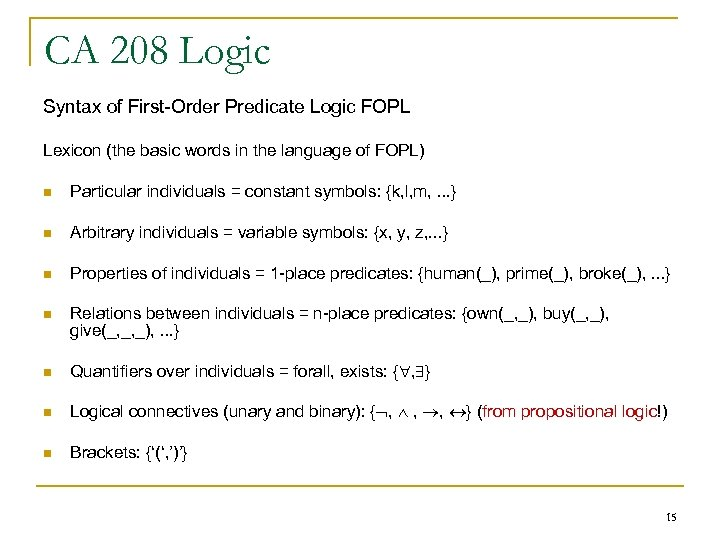CA 208 Logic Syntax of First-Order Predicate Logic FOPL Lexicon (the basic words in the language of FOPL) n Particular individuals = constant symbols: {k, l, m, . . . } n Arbitrary individuals = variable symbols: {x, y, z, . . . } n Properties of individuals = 1 -place predicates: {human(_), prime(_), broke(_), . . . } n Relations between individuals = n-place predicates: {own(_, _), buy(_, _), give(_, _, _), . . . } n Quantifiers over individuals = forall, exists: { , } n Logical connectives (unary and binary): { , , , } (from propositional logic!) n Brackets: {‘(‘, ’)’} 15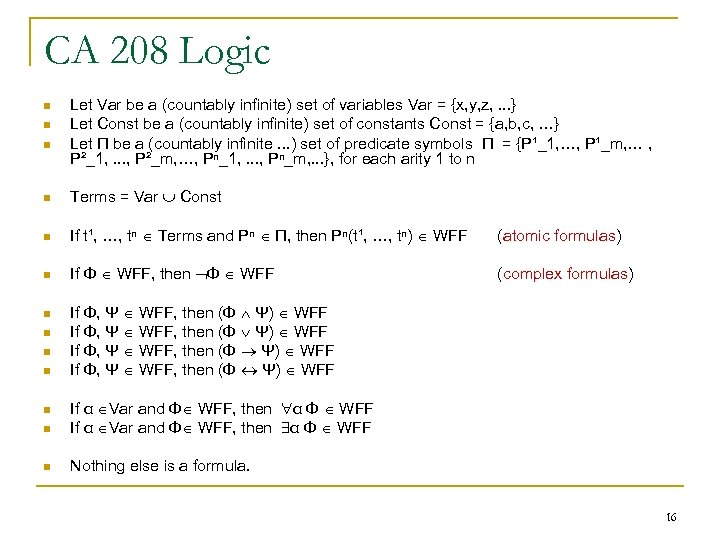CA 208 Logic n n n Let Var be a (countably infinite) set of variables Var = {x, y, z, . . . } Let Const be a (countably infinite) set of constants Const = {a, b, c, …} Let Π be a (countably infinite. . . ) set of predicate symbols Π = {P¹_1, …, P¹_m, … , P²_1, . . . , P²_m, …, Pⁿ_1, . . . , Pⁿ_m, . . . }, for each arity 1 to n n Terms = Var Const n If t¹, …, tⁿ Terms and Pⁿ Π, then Pⁿ(t¹, …, tⁿ) WFF (atomic formulas) n If Φ WFF, then Φ WFF (complex formulas) n n If Φ, Ψ WFF, then (Φ Ψ) WFF n If α Var and Φ WFF, then α Φ WFF n Nothing else is a formula. n 16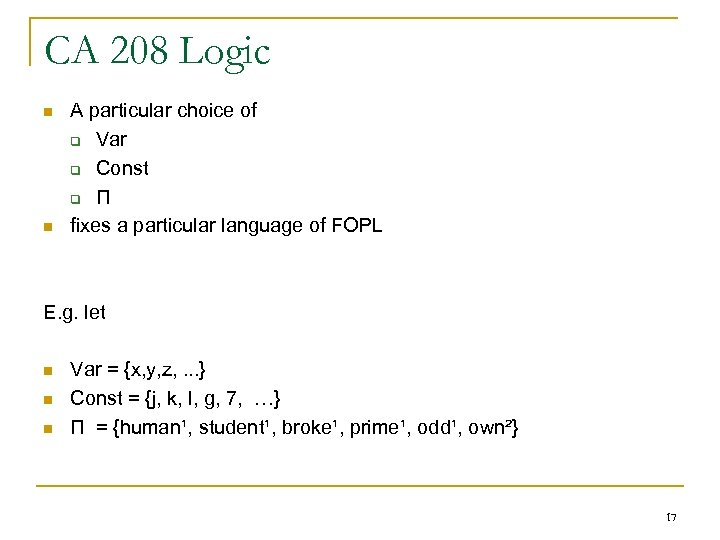CA 208 Logic n n A particular choice of q Var q Const q Π fixes a particular language of FOPL E. g. let n n n Var = {x, y, z, . . . } Const = {j, k, l, g, 7, …} Π = {human¹, student¹, broke¹, prime¹, odd¹, own²} 17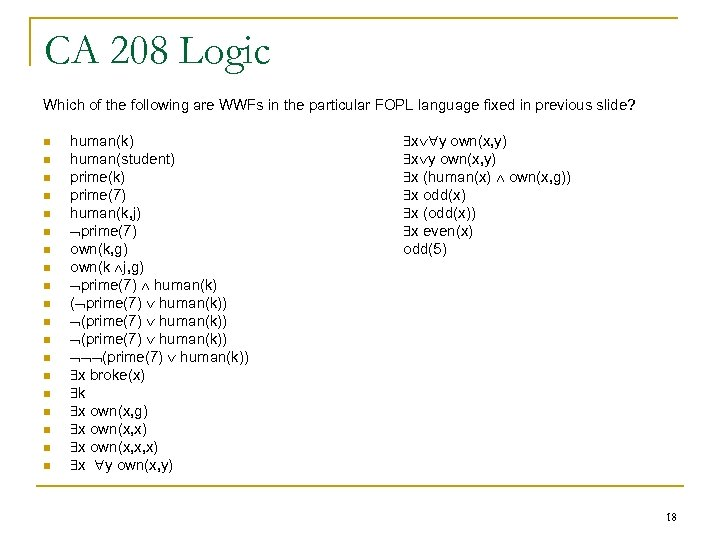CA 208 Logic Which of the following are WWFs in the particular FOPL language fixed in previous slide? n n n n n human(k) human(student) prime(k) prime(7) human(k, j) prime(7) own(k, g) own(k j, g) prime(7) human(k) ( prime(7) human(k)) (prime(7) human(k)) x broke(x) k x own(x, g) x own(x, x, x) x y own(x, y) x (human(x) own(x, g)) x odd(x) x (odd(x)) x even(x) odd(5) 18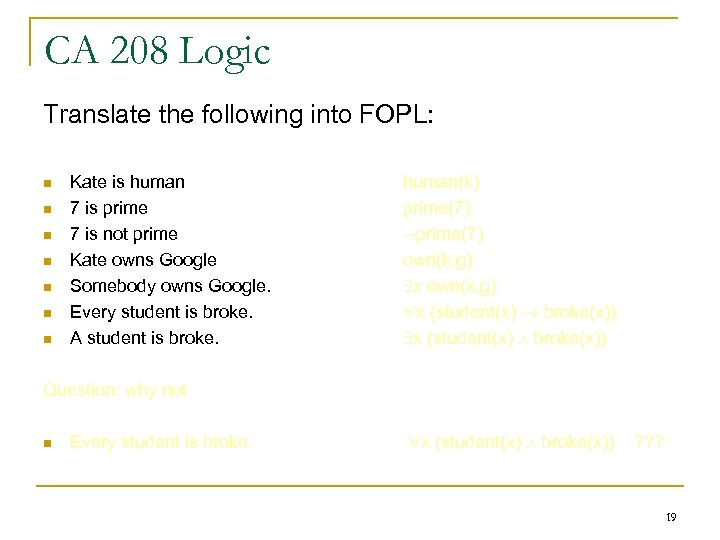CA 208 Logic Translate the following into FOPL: n n n n Kate is human 7 is prime 7 is not prime Kate owns Google Somebody owns Google. Every student is broke. A student is broke. human(k) prime(7) own(k, g) x own(x, g) x (student(x) broke(x)) Question: why not n Every student is broke. x (student(x) broke(x)) ? ? ? 19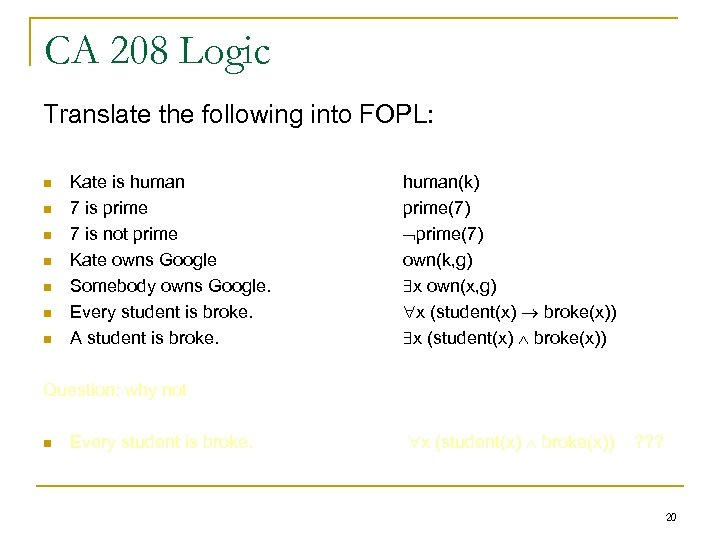CA 208 Logic Translate the following into FOPL: n n n n Kate is human 7 is prime 7 is not prime Kate owns Google Somebody owns Google. Every student is broke. A student is broke. human(k) prime(7) own(k, g) x own(x, g) x (student(x) broke(x)) Question: why not n Every student is broke. x (student(x) broke(x)) ? ? ? 20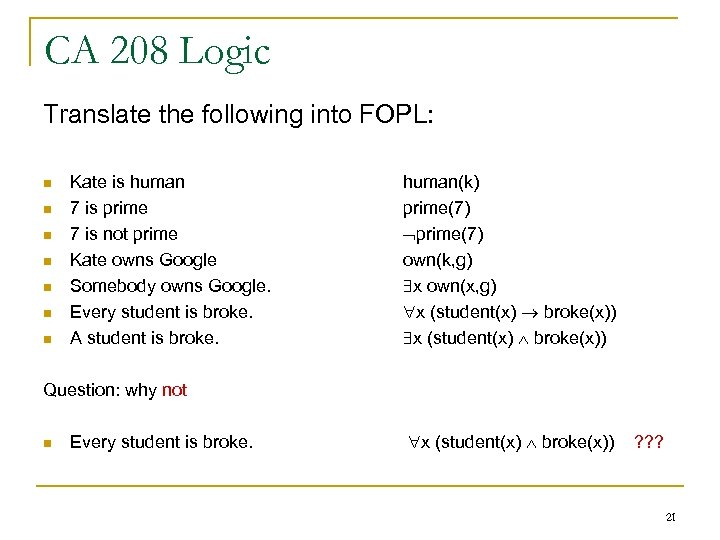CA 208 Logic Translate the following into FOPL: n n n n Kate is human 7 is prime 7 is not prime Kate owns Google Somebody owns Google. Every student is broke. A student is broke. human(k) prime(7) own(k, g) x own(x, g) x (student(x) broke(x)) Question: why not n Every student is broke. x (student(x) broke(x)) ? ? ? 21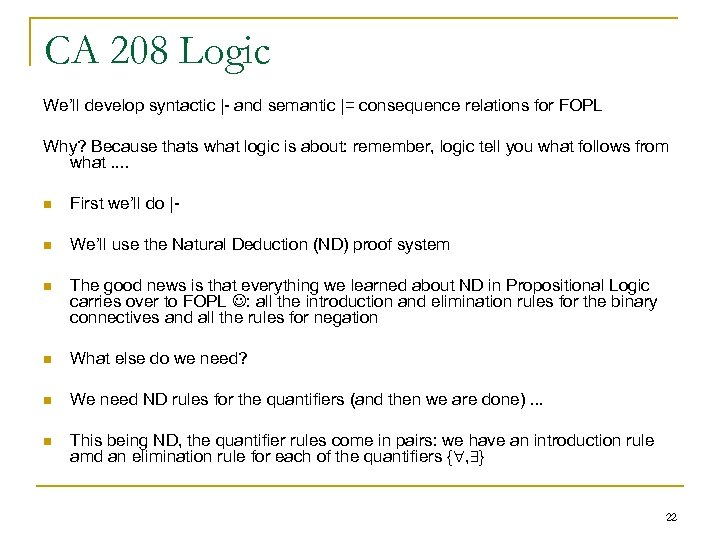CA 208 Logic We’ll develop syntactic |- and semantic |= consequence relations for FOPL Why? Because thats what logic is about: remember, logic tell you what follows from what. . n First we’ll do |- n We’ll use the Natural Deduction (ND) proof system n The good news is that everything we learned about ND in Propositional Logic carries over to FOPL : all the introduction and elimination rules for the binary connectives and all the rules for negation n What else do we need? n We need ND rules for the quantifiers (and then we are done). . . n This being ND, the quantifier rules come in pairs: we have an introduction rule amd an elimination rule for each of the quantifiers { , } 22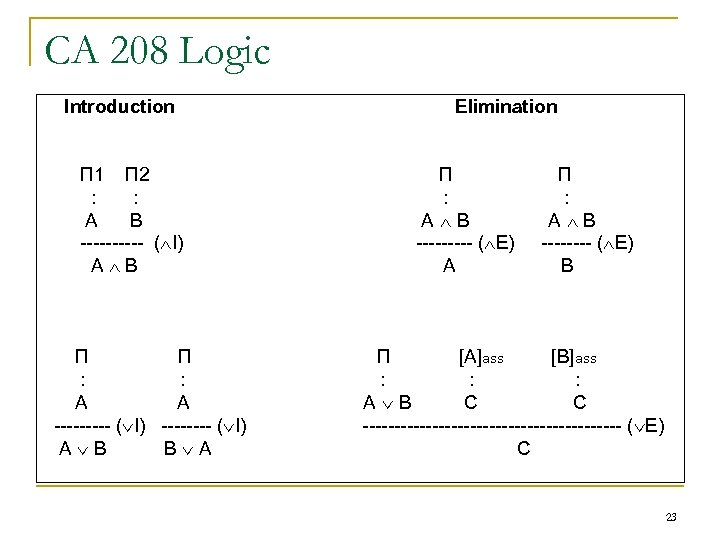CA 208 Logic Introduction П 1 П 2 : : A B ----- ( I) A B П П : : A A ----- ( I) A B B A Elimination П : A B ----- ( E) A П : A B ---- ( E) B П [A]ass [B]ass : : : A B C C --------------------- ( E) C 23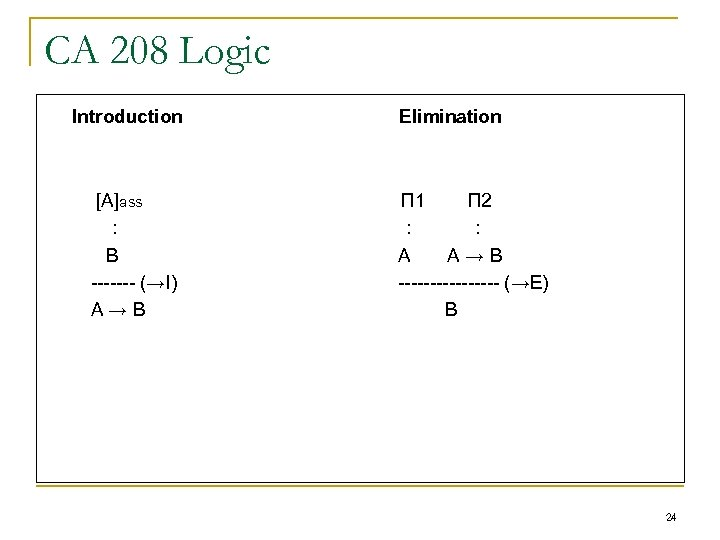CA 208 Logic Introduction [A]ass : B ------- (→I) A→B Elimination П 1 П 2 : : A A→B -------- (→E) B 24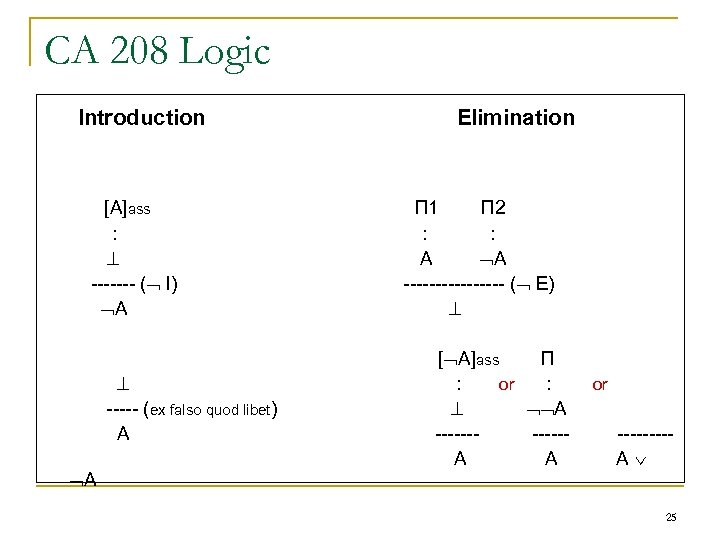CA 208 Logic Introduction [A]ass : ------- ( I) A ----- (ex falso quod libet) A A Elimination П 1 П 2 : : A A -------- ( E) [ A]ass П : or : A ------A A or ----A 25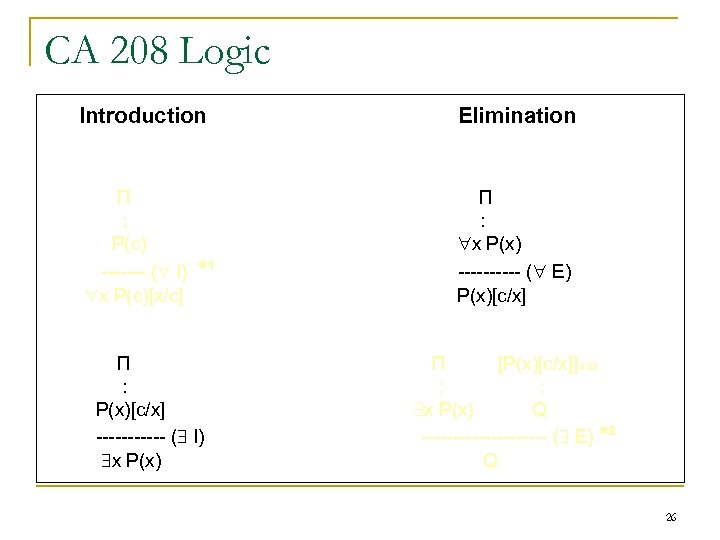CA 208 Logic Introduction П : P(c) ------- ( I) x P(c)[x/c] *¹ П : P(x)[c/x] ------ ( I) x P(x) Elimination П : x P(x) ----- ( E) P(x)[c/x] П [P(x)[c/x]]ass : : x P(x) Q ---------- ( E) *² Q 26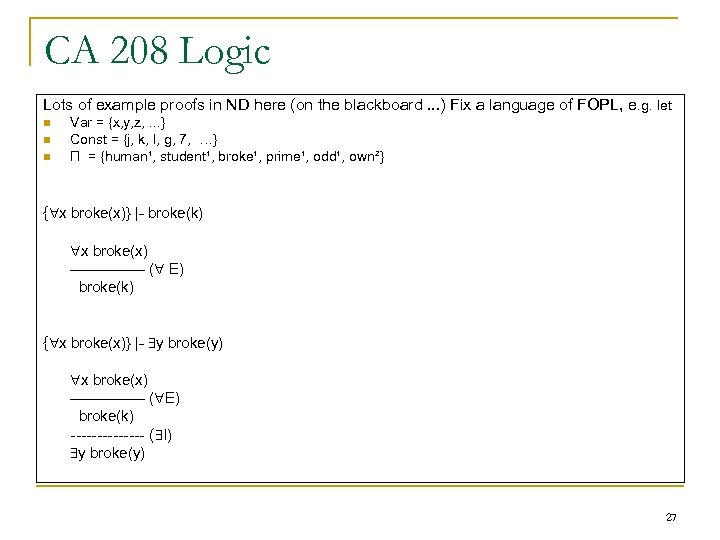CA 208 Logic Lots of example proofs in ND here (on the blackboard. . . ) Fix a language of FOPL, e. g. let n n n Var = {x, y, z, . . . } Const = {j, k, l, g, 7, …} Π = {human¹, student¹, broke¹, prime¹, odd¹, own²} { x broke(x)} |- broke(k) x broke(x) -------- ( E) broke(k) { x broke(x)} |- y broke(y) x broke(x) -------- ( E) broke(k) ------- ( I) y broke(y) 27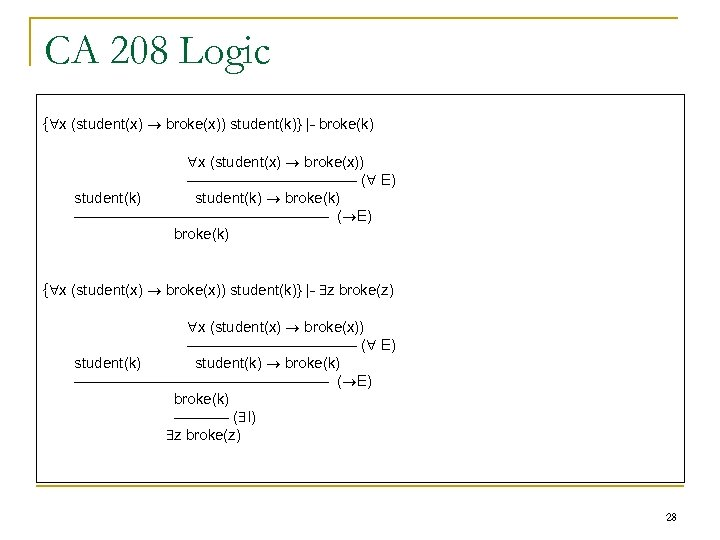CA 208 Logic { x (student(x) broke(x)) student(k)} |- broke(k) x (student(x) broke(x)) ----------------- ( E) student(k) broke(k) -------------------------- ( E) broke(k) { x (student(x) broke(x)) student(k)} |- z broke(z) x (student(x) broke(x)) ----------------- ( E) student(k) broke(k) -------------------------- ( E) broke(k) ------ ( I) z broke(z) 28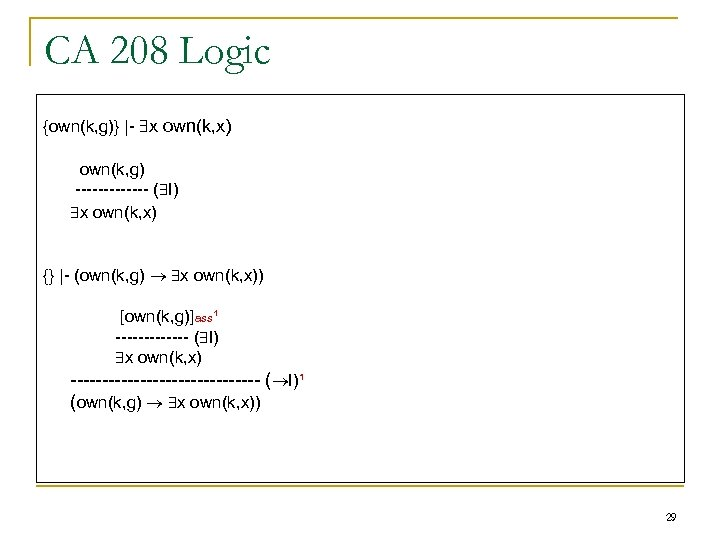CA 208 Logic {own(k, g)} |- x own(k, x) own(k, g) ------- ( I) x own(k, x) {} |- (own(k, g) x own(k, x)) [own(k, g)]ass¹ ------- ( I) x own(k, x) --------------- ( I)¹ (own(k, g) x own(k, x)) 29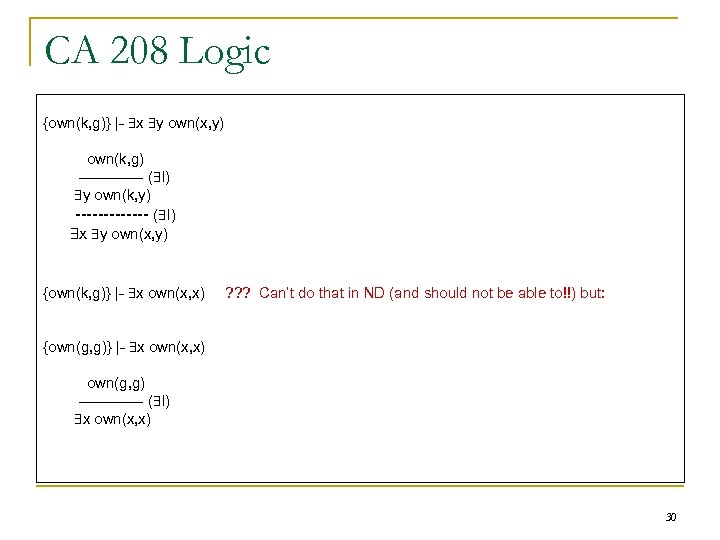CA 208 Logic {own(k, g)} |- x y own(x, y) own(k, g) ------- ( I) y own(k, y) ------- ( I) x y own(x, y) {own(k, g)} |- x own(x, x) ? ? ? Can’t do that in ND (and should not be able to!!) but: {own(g, g)} |- x own(x, x) own(g, g) ------- ( I) x own(x, x) 30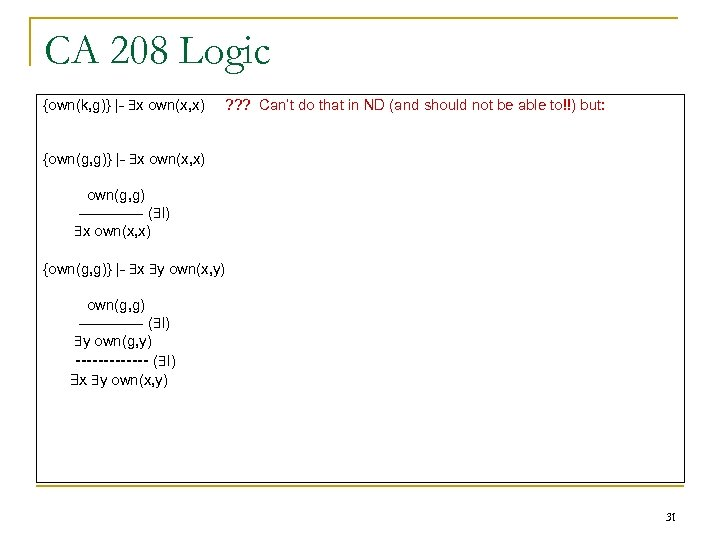CA 208 Logic {own(k, g)} |- x own(x, x) ? ? ? Can’t do that in ND (and should not be able to!!) but: {own(g, g)} |- x own(x, x) own(g, g) ------- ( I) x own(x, x) {own(g, g)} |- x y own(x, y) own(g, g) ------- ( I) y own(g, y) ------- ( I) x y own(x, y) 31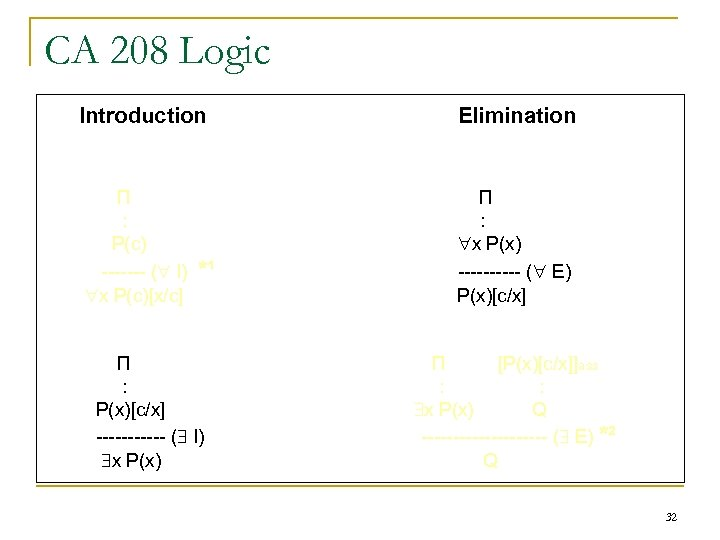CA 208 Logic Introduction П : P(c) ------- ( I) x P(c)[x/c] *¹ П : P(x)[c/x] ------ ( I) x P(x) Elimination П : x P(x) ----- ( E) P(x)[c/x] П [P(x)[c/x]]ass : : x P(x) Q ---------- ( E) *² Q 32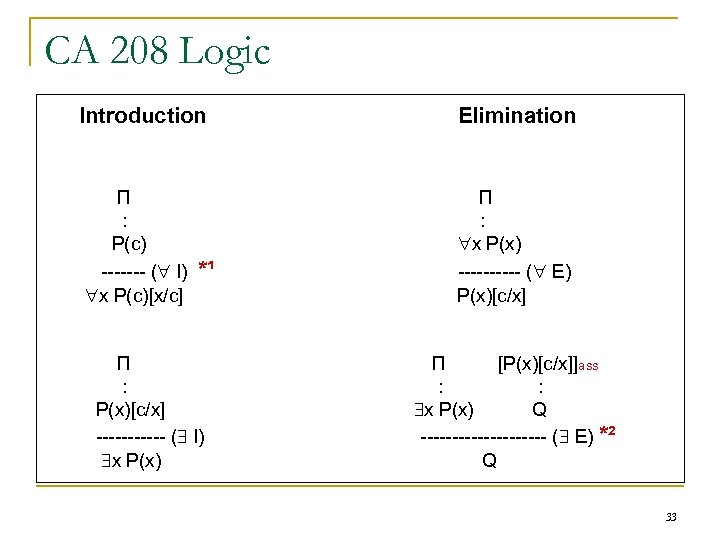CA 208 Logic Introduction П : P(c) ------- ( I) x P(c)[x/c] *¹ П : P(x)[c/x] ------ ( I) x P(x) Elimination П : x P(x) ----- ( E) P(x)[c/x] П [P(x)[c/x]]ass : : x P(x) Q ---------- ( E) *² Q 33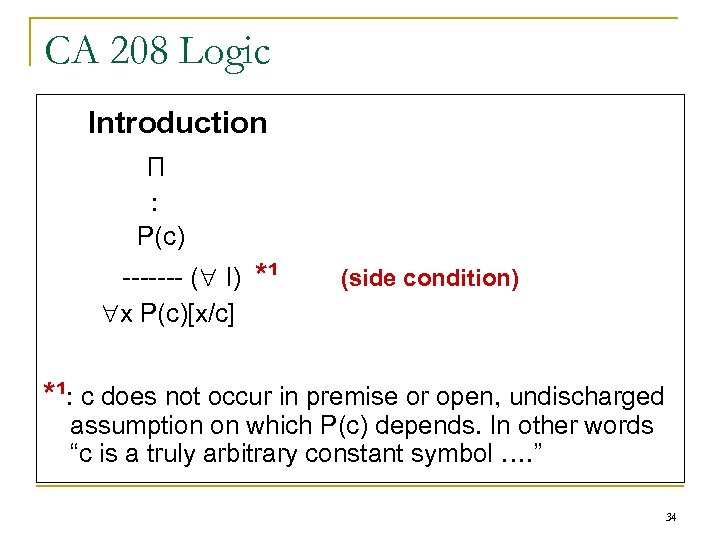CA 208 Logic Introduction П : P(c) ------- ( I) x P(c)[x/c] *¹ (side condition) *¹: c does not occur in premise or open, undischarged assumption on which P(c) depends. In other words “c is a truly arbitrary constant symbol …. ” 34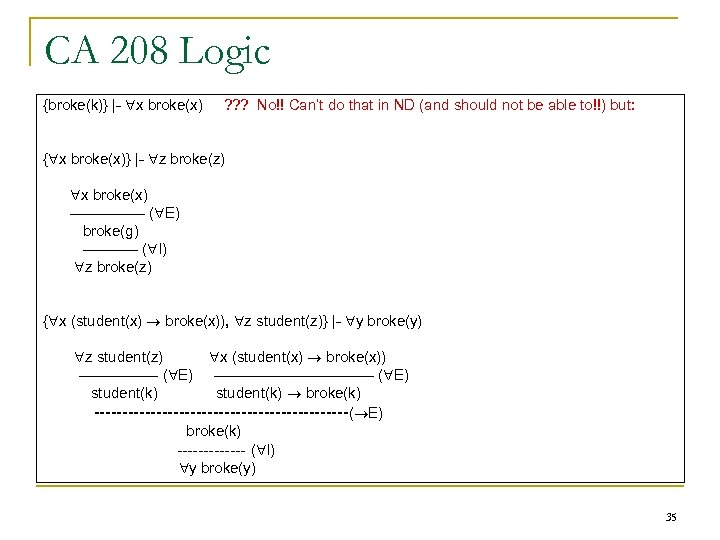CA 208 Logic {broke(k)} |- x broke(x) ? ? ? No!! Can’t do that in ND (and should not be able to!!) but: { x broke(x)} |- z broke(z) x broke(x) -------- ( E) broke(g) ------ ( I) z broke(z) { x (student(x) broke(x)), z student(z)} |- y broke(y) z student(z) -------- ( E) student(k) x (student(x) broke(x)) ---------------- ( E) student(k) broke(k) -----------------------( E) broke(k) ------- ( I) y broke(y) 35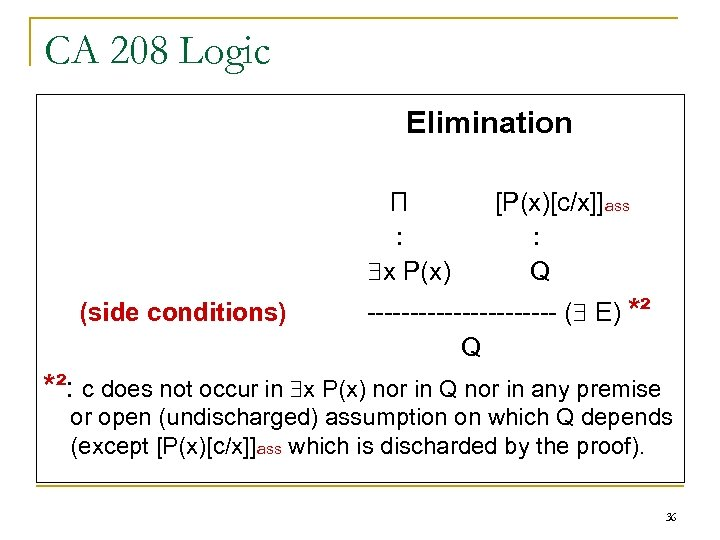CA 208 Logic Elimination П : x P(x) (side conditions) [P(x)[c/x]]ass : Q ----------- ( E) *² Q *²: c does not occur in x P(x) nor in Q nor in any premise or open (undischarged) assumption on which Q depends (except [P(x)[c/x]]ass which is discharded by the proof). 36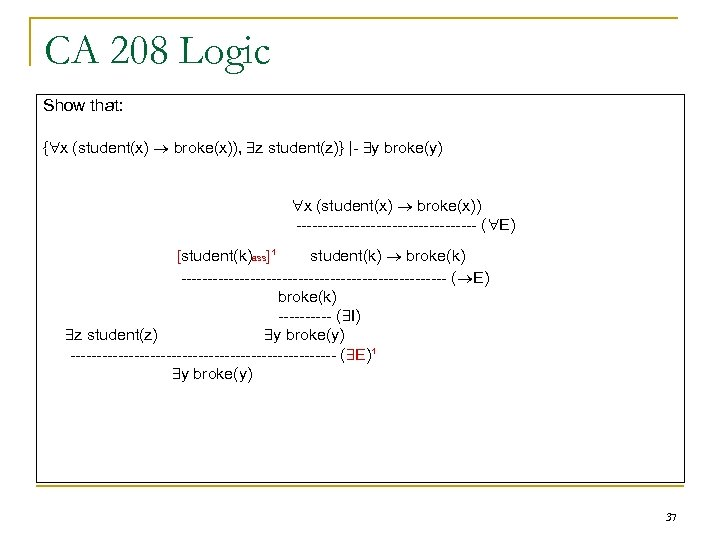CA 208 Logic Show that: { x (student(x) broke(x)), z student(z)} |- y broke(y) x (student(x) broke(x)) ----------------- ( E) [student(k)ass]¹ student(k) broke(k) ------------------------- ( E) broke(k) ----- ( I) z student(z) y broke(y) ------------------------- ( E)¹ y broke(y) 37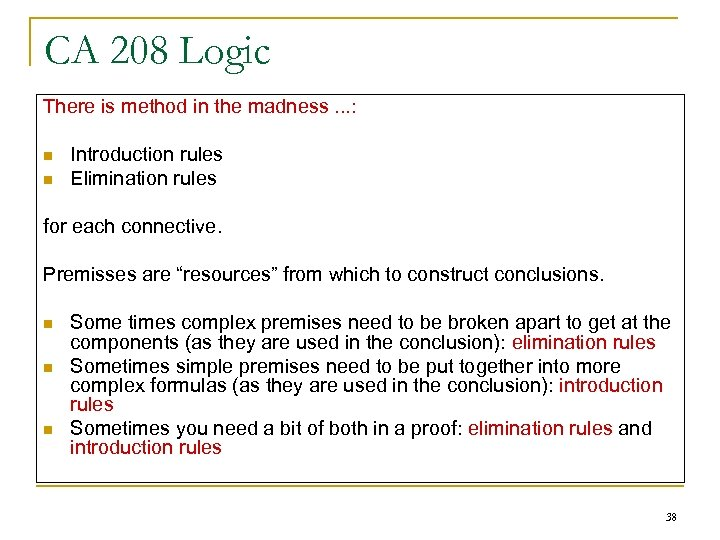CA 208 Logic There is method in the madness. . . : n n Introduction rules Elimination rules for each connective. Premisses are “resources” from which to construct conclusions. n n n Some times complex premises need to be broken apart to get at the components (as they are used in the conclusion): elimination rules Sometimes simple premises need to be put together into more complex formulas (as they are used in the conclusion): introduction rules Sometimes you need a bit of both in a proof: elimination rules and introduction rules 38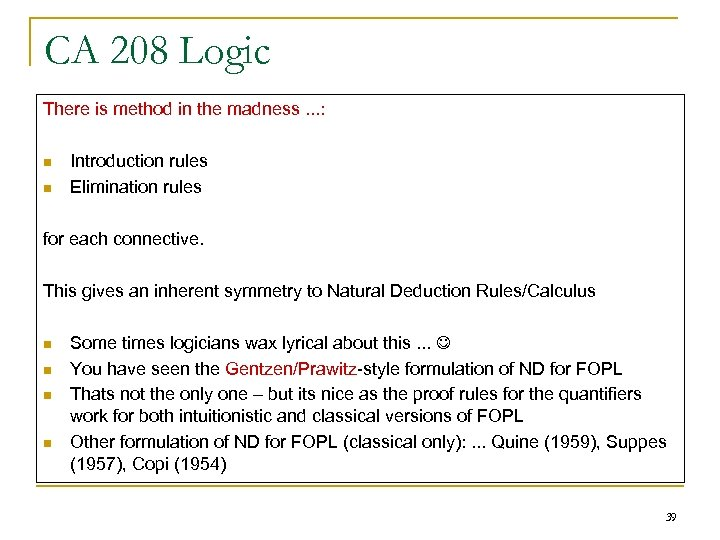CA 208 Logic There is method in the madness. . . : n n Introduction rules Elimination rules for each connective. This gives an inherent symmetry to Natural Deduction Rules/Calculus n n Some times logicians wax lyrical about this. . . You have seen the Gentzen/Prawitz-style formulation of ND for FOPL Thats not the only one – but its nice as the proof rules for the quantifiers work for both intuitionistic and classical versions of FOPL Other formulation of ND for FOPL (classical only): . . . Quine (1959), Suppes (1957), Copi (1954) 39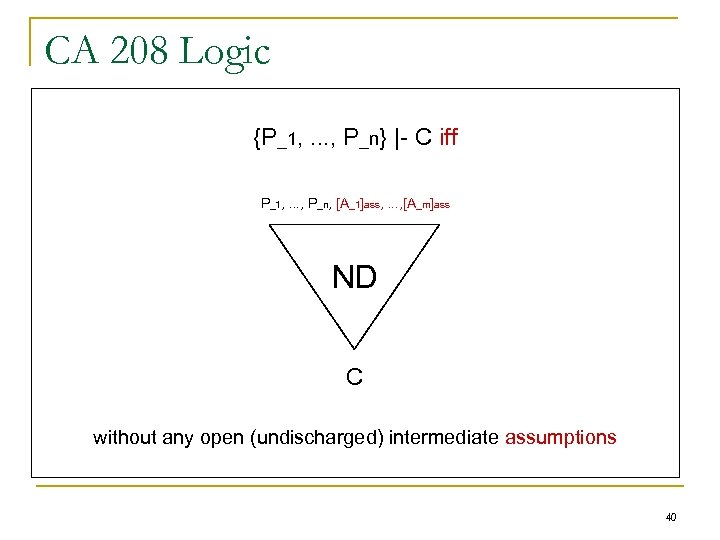CA 208 Logic {P_1, . . . , P_n} |- C iff P_1, . . . , P_n, [A_1]ass, . . . , [A_m]ass ND C without any open (undischarged) intermediate assumptions 40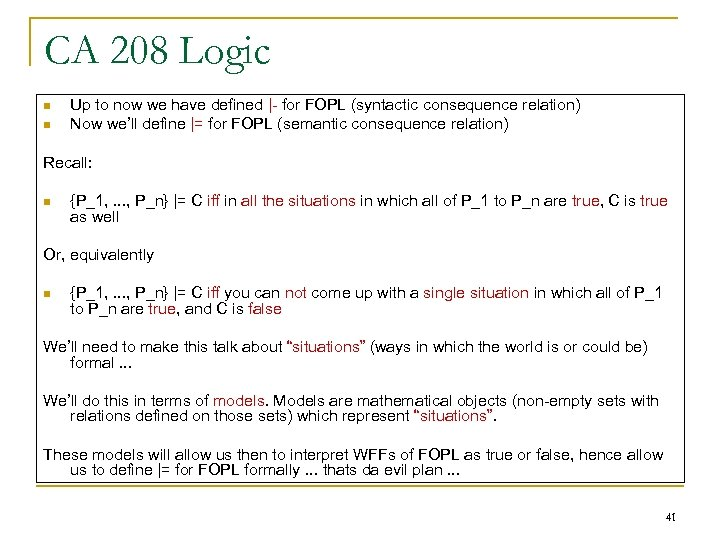CA 208 Logic n n Up to now we have defined |- for FOPL (syntactic consequence relation) Now we’ll define |= for FOPL (semantic consequence relation) Recall: n {P_1, . . . , P_n} |= C iff in all the situations in which all of P_1 to P_n are true, C is true as well Or, equivalently n {P_1, . . . , P_n} |= C iff you can not come up with a single situation in which all of P_1 to P_n are true, and C is false We’ll need to make this talk about “situations” (ways in which the world is or could be) formal. . . We’ll do this in terms of models. Models are mathematical objects (non-empty sets with relations defined on those sets) which represent “situations”. These models will allow us then to interpret WFFs of FOPL as true or false, hence allow us to define |= for FOPL formally. . . thats da evil plan. . . 41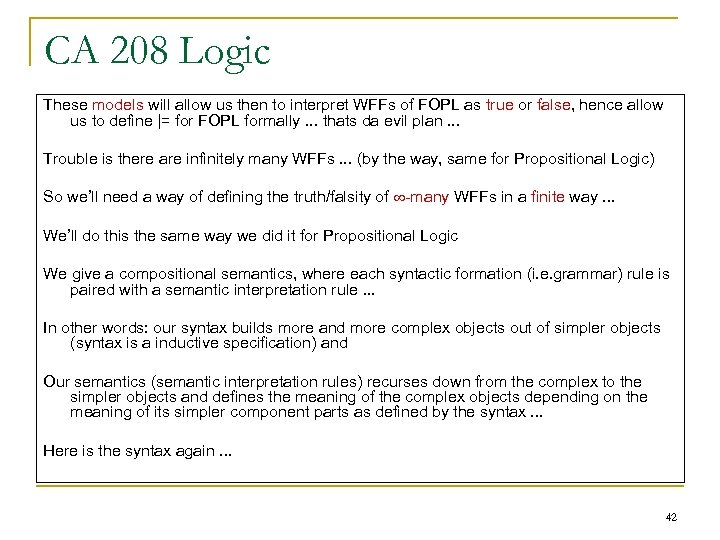CA 208 Logic These models will allow us then to interpret WFFs of FOPL as true or false, hence allow us to define |= for FOPL formally. . . thats da evil plan. . . Trouble is there are infinitely many WFFs. . . (by the way, same for Propositional Logic) So we’ll need a way of defining the truth/falsity of -many WFFs in a finite way. . . We’ll do this the same way we did it for Propositional Logic We give a compositional semantics, where each syntactic formation (i. e. grammar) rule is paired with a semantic interpretation rule. . . In other words: our syntax builds more and more complex objects out of simpler objects (syntax is a inductive specification) and Our semantics (semantic interpretation rules) recurses down from the complex to the simpler objects and defines the meaning of the complex objects depending on the meaning of its simpler component parts as defined by the syntax. . . Here is the syntax again. . . 42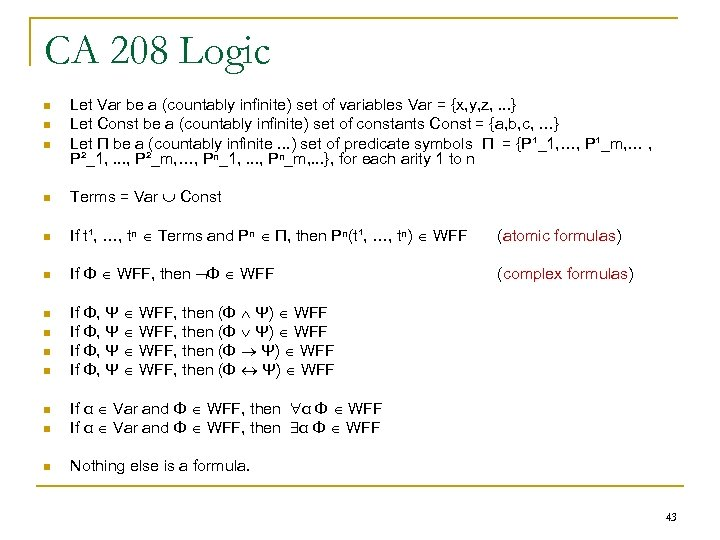CA 208 Logic n n n Let Var be a (countably infinite) set of variables Var = {x, y, z, . . . } Let Const be a (countably infinite) set of constants Const = {a, b, c, …} Let Π be a (countably infinite. . . ) set of predicate symbols Π = {P¹_1, …, P¹_m, … , P²_1, . . . , P²_m, …, Pⁿ_1, . . . , Pⁿ_m, . . . }, for each arity 1 to n n Terms = Var Const n If t¹, …, tⁿ Terms and Pⁿ Π, then Pⁿ(t¹, …, tⁿ) WFF (atomic formulas) n If Φ WFF, then Φ WFF (complex formulas) n n If Φ, Ψ WFF, then (Φ Ψ) WFF n If α Var and Φ WFF, then α Φ WFF n Nothing else is a formula. n 43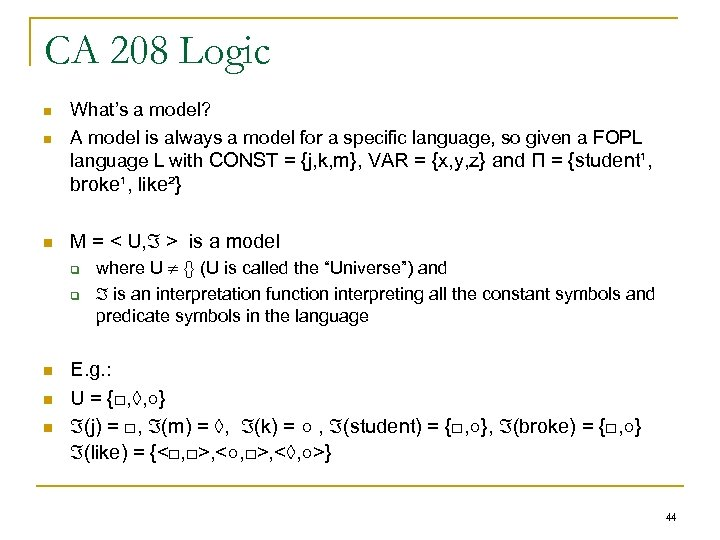CA 208 Logic n n What’s a model? A model is always a model for a specific language, so given a FOPL language L with CONST = {j, k, m}, VAR = {x, y, z} and П = {student¹, broke¹, like²} n M = < U, > is a model q q n n n where U {} (U is called the “Universe”) and is an interpretation function interpreting all the constant symbols and predicate symbols in the language E. g. : U = {□, ◊, ○} (j) = □, (m) = ◊, (k) = ○ , (student) = {□, ○}, (broke) = {□, ○} (like) = {<□, □>, <○, □>, <◊, ○>} 44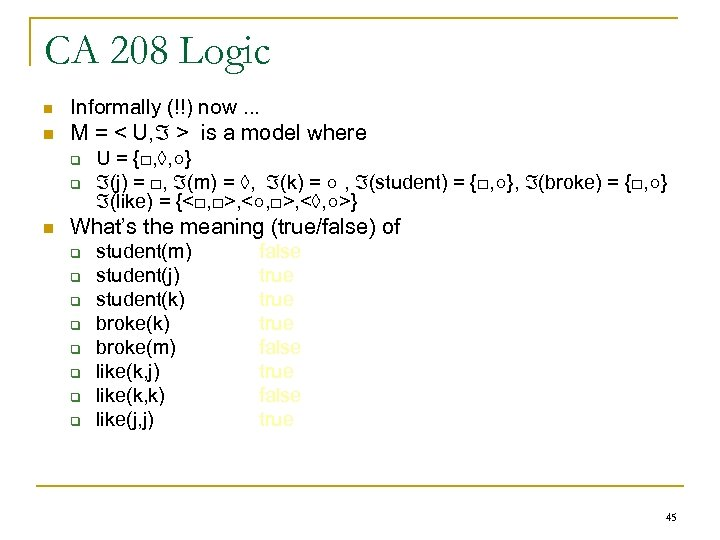CA 208 Logic n n n Informally (!!) now. . . M = < U, > is a model where q U = {□, ◊, ○} q (j) = □, (m) = ◊, (k) = ○ , (student) = {□, ○}, (broke) = {□, ○} (like) = {<□, □>, <○, □>, <◊, ○>} What’s the meaning (true/false) of q student(m) false q student(j) true q student(k) true q broke(m) false q like(k, j) true q like(k, k) false q like(j, j) true 45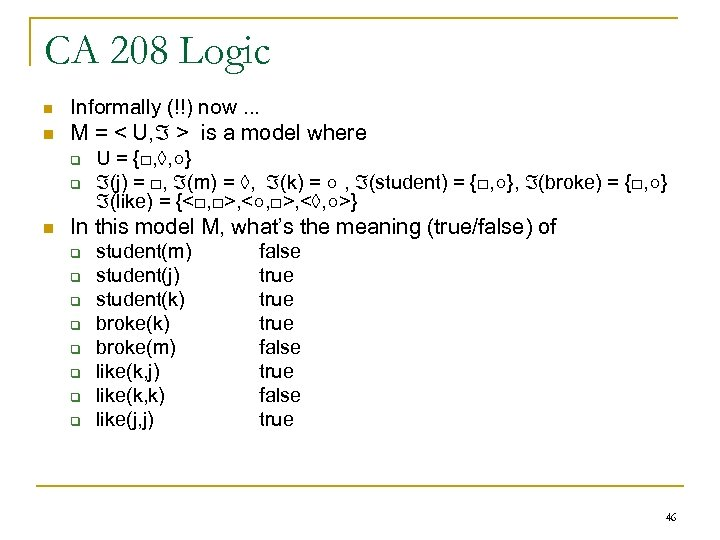CA 208 Logic n n n Informally (!!) now. . . M = < U, > is a model where q U = {□, ◊, ○} q (j) = □, (m) = ◊, (k) = ○ , (student) = {□, ○}, (broke) = {□, ○} (like) = {<□, □>, <○, □>, <◊, ○>} In this model M, what’s the meaning (true/false) of q student(m) false q student(j) true q student(k) true q broke(m) false q like(k, j) true q like(k, k) false q like(j, j) true 46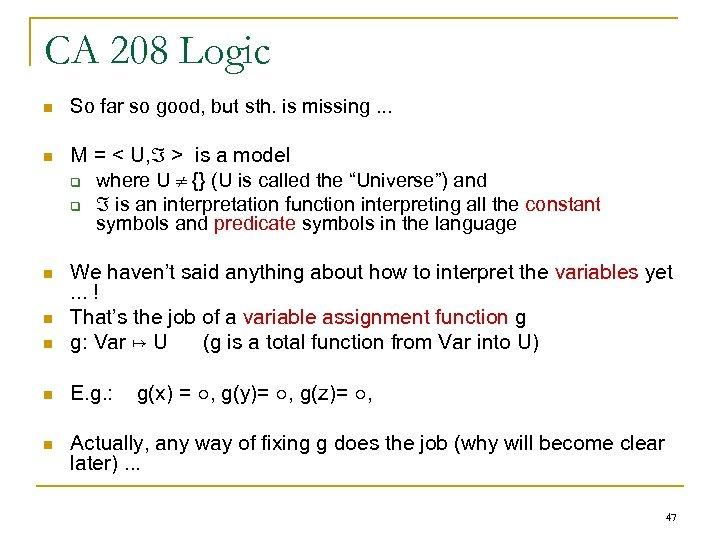CA 208 Logic n So far so good, but sth. is missing. . . n M = < U, > is a model q where U {} (U is called the “Universe”) and q is an interpretation function interpreting all the constant symbols and predicate symbols in the language n n We haven’t said anything about how to interpret the variables yet. . . ! That’s the job of a variable assignment function g g: Var ↦ U (g is a total function from Var into U) n E. g. : n Actually, any way of fixing g does the job (why will become clear later). . . n g(x) = ○, g(y)= ○, g(z)= ○, 47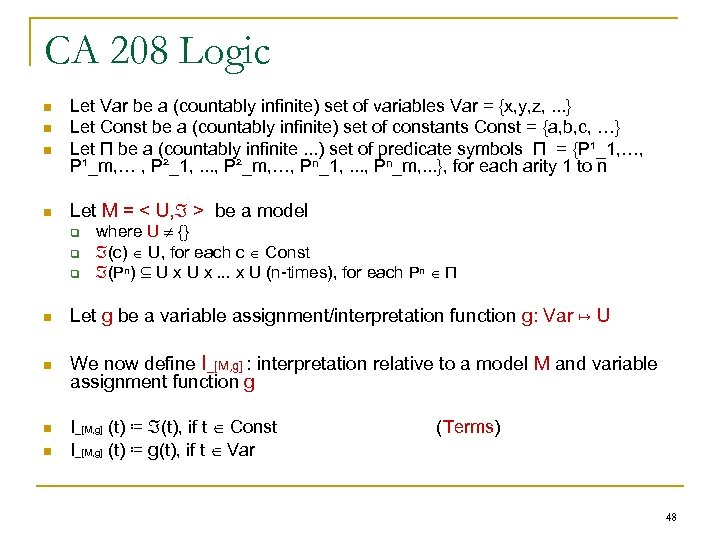CA 208 Logic n n Let Var be a (countably infinite) set of variables Var = {x, y, z, . . . } Let Const be a (countably infinite) set of constants Const = {a, b, c, …} Let Π be a (countably infinite. . . ) set of predicate symbols Π = {P¹_1, …, P¹_m, … , P²_1, . . . , P²_m, …, Pⁿ_1, . . . , Pⁿ_m, . . . }, for each arity 1 to n Let M = < U, > be a model q q q where U {} (c) U, for each c Const (Pⁿ) ⊆ U x. . . x U (n-times), for each Pⁿ Π n Let g be a variable assignment/interpretation function g: Var ↦ U n We now define I_[M, g] : interpretation relative to a model M and variable assignment function g n n I_[M, g] (t) ≔ (t), if t Const I_[M, g] (t) ≔ g(t), if t Var (Terms) 48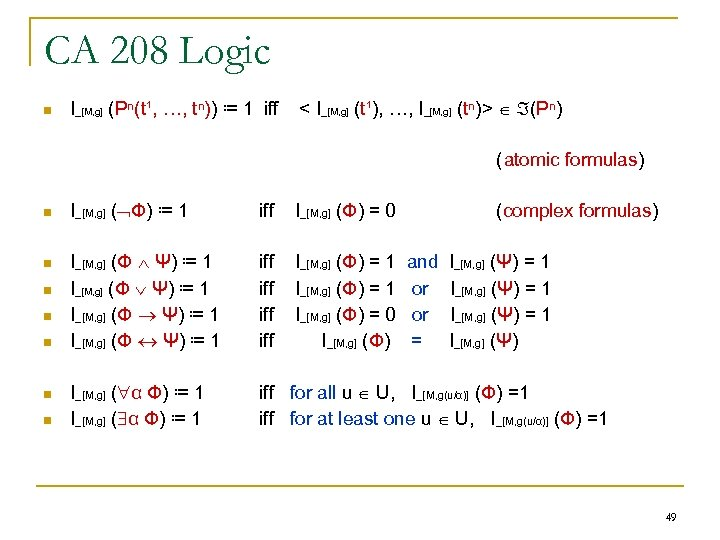CA 208 Logic n I_[M, g] (Pⁿ(t¹, …, tⁿ)) ≔ 1 iff < I_[M, g] (t¹), …, I_[M, g] (tⁿ)> (Pⁿ) (atomic formulas) n n n n I_[M, g] ( Φ) ≔ 1 iff I_[M, g] (Φ) = 0 I_[M, g] (Φ Ψ) ≔ 1 iff iff I_[M, g] (Φ) = 1 I_[M, g] (Φ) = 0 I_[M, g] (Φ) I_[M, g] ( α Φ) ≔ 1 iff for all u U, I_[M, g(u/α)] (Φ) =1 iff for at least one u U, I_[M, g(u/α)] (Φ) =1 (complex formulas) and or or = I_[M, g] (Ψ) = 1 I_[M, g] (Ψ) 49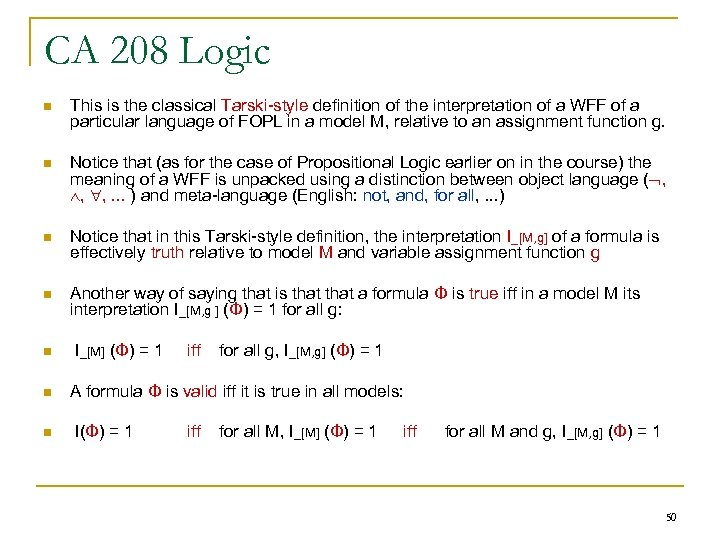CA 208 Logic n This is the classical Tarski-style definition of the interpretation of a WFF of a particular language of FOPL in a model M, relative to an assignment function g. n Notice that (as for the case of Propositional Logic earlier on in the course) the meaning of a WFF is unpacked using a distinction between object language ( , , , . . . ) and meta-language (English: not, and, for all, . . . ) n Notice that in this Tarski-style definition, the interpretation I_[M, g] of a formula is effectively truth relative to model M and variable assignment function g n Another way of saying that is that a formula Φ is true iff in a model M its interpretation I_[M, g ] (Φ) = 1 for all g: n n n I_[M] (Φ) = 1 iff for all g, I_[M, g] (Φ) = 1 A formula Φ is valid iff it is true in all models: I(Φ) = 1 iff for all M, I_[M] (Φ) = 1 iff for all M and g, I_[M, g] (Φ) = 1 50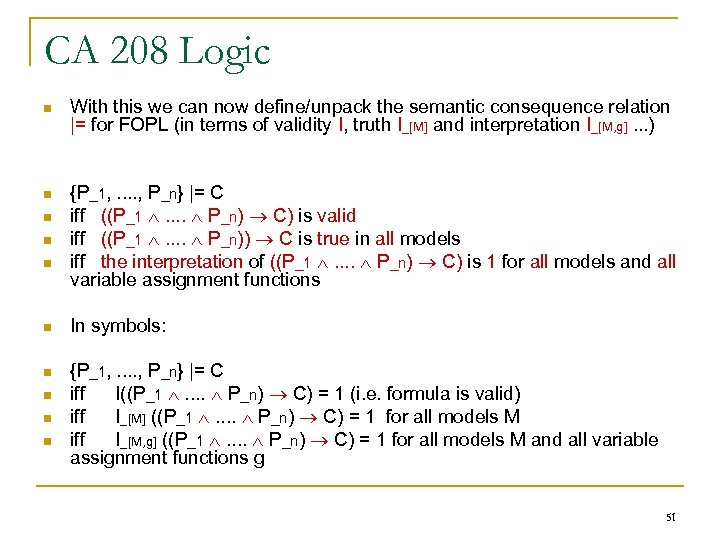CA 208 Logic n With this we can now define/unpack the semantic consequence relation |= for FOPL (in terms of validity I, truth I_[M] and interpretation I_[M, g]. . . ) n {P_1, . . , P_n} |= C iff ((P_1 . . P_n) C) is valid iff ((P_1 . . P_n)) C is true in all models iff the interpretation of ((P_1 . . P_n) C) is 1 for all models and all variable assignment functions n n In symbols: n {P_1, . . , P_n} |= C iff I((P_1 . . P_n) C) = 1 (i. e. formula is valid) iff I_[M] ((P_1 . . P_n) C) = 1 for all models M iff I_[M, g] ((P_1 . . P_n) C) = 1 for all models M and all variable assignment functions g n n n 51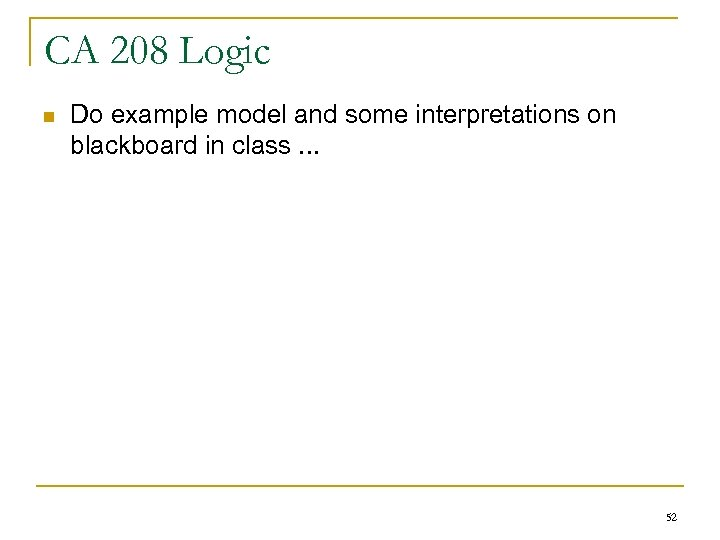CA 208 Logic n Do example model and some interpretations on blackboard in class. . . 52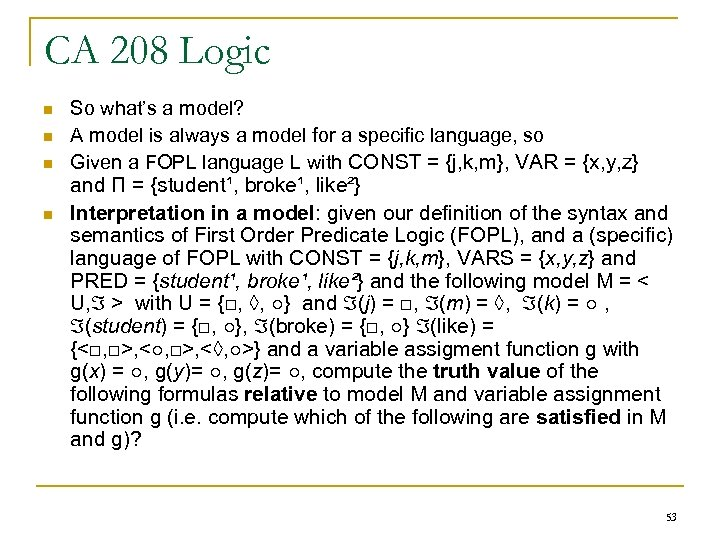CA 208 Logic n n So what’s a model? A model is always a model for a specific language, so Given a FOPL language L with CONST = {j, k, m}, VAR = {x, y, z} and П = {student¹, broke¹, like²} Interpretation in a model: given our definition of the syntax and semantics of First Order Predicate Logic (FOPL), and a (specific) language of FOPL with CONST = {j, k, m}, VARS = {x, y, z} and PRED = {student¹, broke¹, like²} and the following model M = < U, > with U = {□, ◊, ○} and (j) = □, (m) = ◊, (k) = ○ , (student) = {□, ○}, (broke) = {□, ○} (like) = {<□, □>, <○, □>, <◊, ○>} and a variable assigment function g with g(x) = ○, g(y)= ○, g(z)= ○, compute the truth value of the following formulas relative to model M and variable assignment function g (i. e. compute which of the following are satisfied in M and g)? 53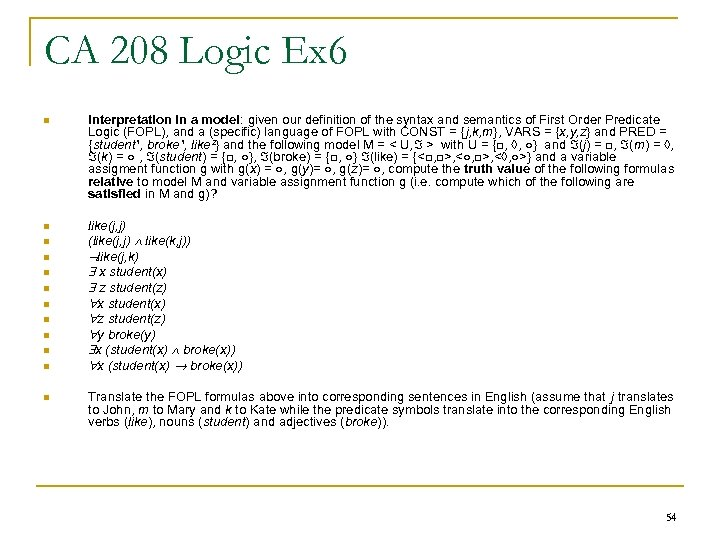CA 208 Logic Ex 6 n Interpretation in a model: given our definition of the syntax and semantics of First Order Predicate Logic (FOPL), and a (specific) language of FOPL with CONST = {j, k, m}, VARS = {x, y, z} and PRED = {student¹, broke¹, like²} and the following model M = < U, > with U = {□, ◊, ○} and (j) = □, (m) = ◊, (k) = ○ , (student) = {□, ○}, (broke) = {□, ○} (like) = {<□, □>, <○, □>, <◊, ○>} and a variable assigment function g with g(x) = ○, g(y)= ○, g(z)= ○, compute the truth value of the following formulas relative to model M and variable assignment function g (i. e. compute which of the following are satisfied in M and g)? n like(j, j) (like(j, j) like(k, j)) like(j, k) x student(x) z student(z) x student(x) z student(z) y broke(y) x (student(x) broke(x)) n n n n n Translate the FOPL formulas above into corresponding sentences in English (assume that j translates to John, m to Mary and k to Kate while the predicate symbols translate into the corresponding English verbs (like), nouns (student) and adjectives (broke)). 54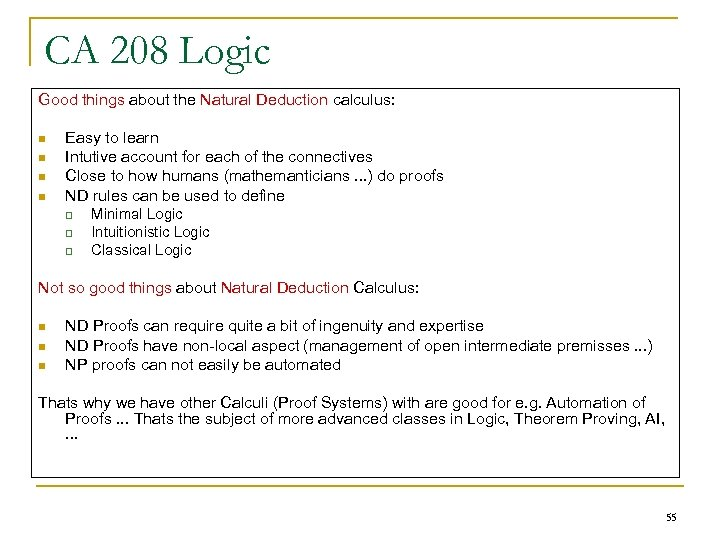CA 208 Logic Good things about the Natural Deduction calculus: n n Easy to learn Intutive account for each of the connectives Close to how humans (mathemanticians. . . ) do proofs ND rules can be used to define q q q Minimal Logic Intuitionistic Logic Classical Logic Not so good things about Natural Deduction Calculus: n n n ND Proofs can require quite a bit of ingenuity and expertise ND Proofs have non-local aspect (management of open intermediate premisses. . . ) NP proofs can not easily be automated Thats why we have other Calculi (Proof Systems) with are good for e. g. Automation of Proofs. . . Thats the subject of more advanced classes in Logic, Theorem Proving, AI, . . . 55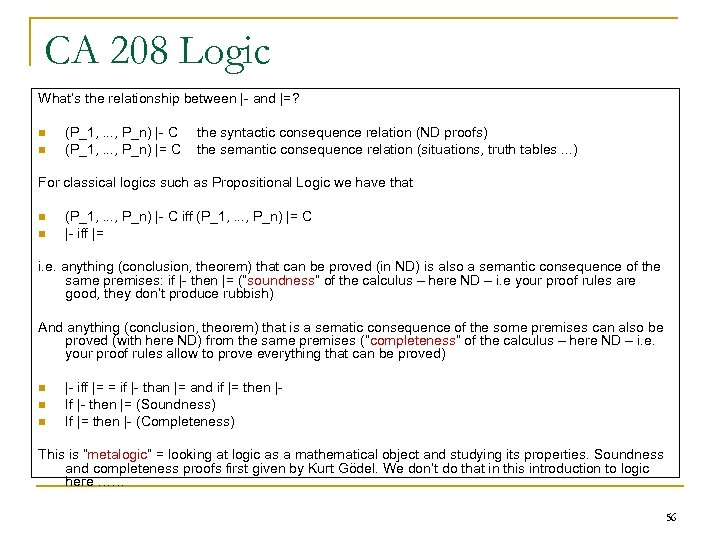CA 208 Logic What’s the relationship between |- and |=? n n (P_1, . . . , P_n) |- C (P_1, . . . , P_n) |= C the syntactic consequence relation (ND proofs) the semantic consequence relation (situations, truth tables. . . ) For classical logics such as Propositional Logic we have that n n (P_1, . . . , P_n) |- C iff (P_1, . . . , P_n) |= C |- iff |= i. e. anything (conclusion, theorem) that can be proved (in ND) is also a semantic consequence of the same premises: if |- then |= (“soundness” of the calculus – here ND – i. e your proof rules are good, they don’t produce rubbish) And anything (conclusion, theorem) that is a sematic consequence of the some premises can also be proved (with here ND) from the same premises (“completeness” of the calculus – here ND – i. e. your proof rules allow to prove everything that can be proved) n n n |- iff |= = if |- than |= and if |= then |If |- then |= (Soundness) If |= then |- (Completeness) This is “metalogic” = looking at logic as a mathematical object and studying its properties. Soundness and completeness proofs first given by Kurt Gödel. We don’t do that in this introduction to logic here …… 56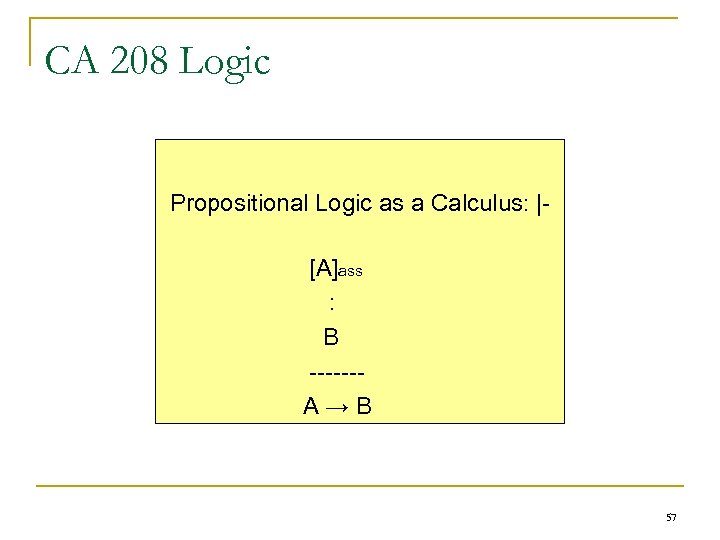CA 208 Logic Propositional Logic as a Calculus: |[A]ass : B ------A→B 57